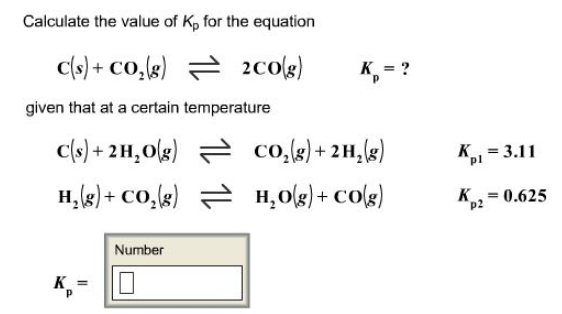Chemistry Practice Problems Equilibrium Expressions Practice Problems Solution: Calculate the value of Kp for the equation C (s) +...

# Solution: Calculate the value of Kp for the equation C (s) + CO2 (g) ⇌ 2CO (g)                       Kp = ? given that at a certain temperature C (s) + 2H2O (g) ⇌ CO2 (g) + 2H2 (g)     Kp1 = 3.11 H2 (s) + CO2 (g) ⇌ H2O(g) + CO(g)        Kp2 = 0.625

###### Problem

Calculate the value of Kp for the equation

C (s) + CO2 (g) ⇌ 2CO (g)                       Kp = ?

given that at a certain temperature

C (s) + 2H2O (g) ⇌ CO(g) + 2H2 (g)     Kp1 = 3.11

H2 (s) + CO2 (g) ⇌ H2O(g) + CO(g)        Kp2 = 0.625View Complete Written Solution

Equilibrium Expressions

Equilibrium Expressions

#### Q. Phosgene (carbonyl chloride), COCl2, is an extremely toxic gas that is used in manufacturing certain dyes and plastics. Phosgene can be produced by re...

Solved • Mon Sep 17 2018 18:51:01 GMT-0400 (EDT)

Equilibrium Expressions

#### Q. For the reaction shown here 2NH3 (g) ⇌ 3 H2 (g) + N2 (g) the equilibrium concentrations were found to be [NH3] = 0.250 M [H2] = 0.540 M [N2] = 0.800 M...

Solved • Fri Sep 07 2018 12:21:22 GMT-0400 (EDT)

Equilibrium Expressions

#### Q. Write the following equilibrium-constant expressions: (a) Kc for Cr (s) + 3Ag+ (aq) ⇌ Cr3+ (aq) + 3Ag (s), (b) Kp for 3Fe (s) + 4H2O(g) ⇌ Fe3O4 (s) + ...

Solved • Wed Sep 05 2018 17:19:58 GMT-0400 (EDT)

Equilibrium Expressions

#### Q. Consider the following elementary reaction: ICl (g) + H2 (g) → HI (g) + HCl (g) Suppose we let k1 stand for the rate constant of this reaction, and k-...

Solved • Wed Sep 05 2018 11:31:22 GMT-0400 (EDT)